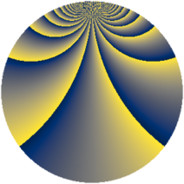# Properties

 Label 1152.3.btLevel $1152$ Weight $3$ Character orbit 1152.bt Rep. character $\chi_{1152}(5,\cdot)$ Character field $\Q(\zeta_{96})$ Dimension $12224$ Sturm bound $576$

# Learn more

## Defining parameters

 Level: $$N$$ $$=$$ $$1152 = 2^{7} \cdot 3^{2}$$ Weight: $$k$$ $$=$$ $$3$$ Character orbit: $$[\chi]$$ $$=$$ 1152.bt (of order $$96$$ and degree $$32$$) Character conductor: $$\operatorname{cond}(\chi)$$ $$=$$ $$1152$$ Character field: $$\Q(\zeta_{96})$$ Sturm bound: $$576$$

## Dimensions

The following table gives the dimensions of various subspaces of $$M_{3}(1152, [\chi])$$.

Total New Old
Modular forms 12352 12352 0
Cusp forms 12224 12224 0
Eisenstein series 128 128 0

## Trace form

 $$12224q - 48q^{2} - 32q^{3} - 16q^{4} - 48q^{5} - 32q^{6} - 16q^{7} - 32q^{9} + O(q^{10})$$ $$12224q - 48q^{2} - 32q^{3} - 16q^{4} - 48q^{5} - 32q^{6} - 16q^{7} - 32q^{9} - 64q^{10} - 48q^{11} - 32q^{12} - 16q^{13} - 48q^{14} - 32q^{15} - 16q^{16} - 32q^{18} - 64q^{19} - 48q^{20} - 32q^{21} - 16q^{22} - 48q^{23} - 32q^{24} - 16q^{25} - 32q^{27} - 64q^{28} - 48q^{29} - 32q^{30} - 16q^{31} - 48q^{32} - 32q^{33} - 16q^{34} - 32q^{36} - 64q^{37} - 48q^{38} - 32q^{39} - 16q^{40} - 48q^{41} - 32q^{42} - 16q^{43} - 32q^{45} - 64q^{46} - 48q^{47} - 32q^{48} - 16q^{49} - 48q^{50} - 32q^{51} - 16q^{52} - 32q^{54} - 64q^{55} - 48q^{56} - 32q^{57} + 704q^{58} - 48q^{59} - 32q^{60} - 16q^{61} - 64q^{64} - 32q^{66} - 16q^{67} - 48q^{68} - 32q^{69} - 16q^{70} - 32q^{72} - 64q^{73} - 48q^{74} - 32q^{75} + 1232q^{76} - 48q^{77} - 32q^{78} - 16q^{79} - 32q^{81} - 64q^{82} - 48q^{83} - 32q^{84} - 16q^{85} - 48q^{86} - 32q^{87} - 16q^{88} - 32q^{90} - 64q^{91} - 48q^{92} - 32q^{93} - 16q^{94} - 48q^{95} - 32q^{96} - 16q^{97} - 32q^{99} + O(q^{100})$$

## Decomposition of $$S_{3}^{\mathrm{new}}(1152, [\chi])$$ into newform subspaces

The newforms in this space have not yet been added to the LMFDB.